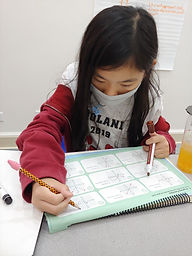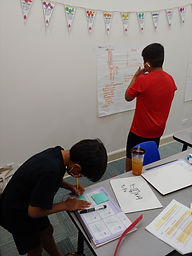## Ms. Mari

### Target 1​

###### Lesson Type:

New

Algebra

:

Function

Graphically represent function equations in the coordinate plane.

###### 1:

Distinguish between functions and non-functions.

###### 2:

Justify whether a graphic representation is a function.

8th

###### Vocabulary:

FUNCTION, VERTICAL LINE TEST

Activities:

- Determined whether or not a relationship was a function using the "vertical line test".

- Sorted tables, graphs, ordered pairs into two categories: Function or Not a Function.### Home Exploration

###### Guiding Questions:## Absent Students:

### Target 2

:

###### 1:

Identify the equation y = mx + b as defining a linear function which graphs a straight line.

7th

###### Vocabulary:

RATE OF CHANGE, Y-INTERCEPT

Activities:

- Calculated the rate of change given a set of inputs and outputs.

- Used the rate of change to create an equation defining the relationship between inputs and outputs.

- Revisited previous activity with proportional and nonproportional relationships and created equations for those situations.### Home Exploration

###### Guiding Questions:### Target 3

:

###### Vocabulary:

Activities:### Home Exploration